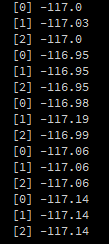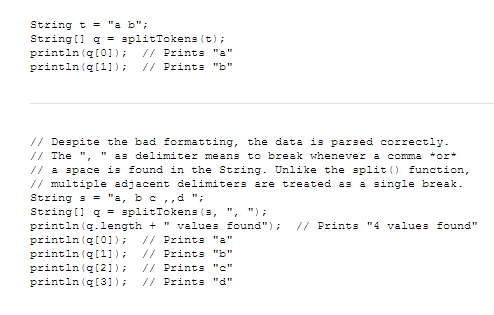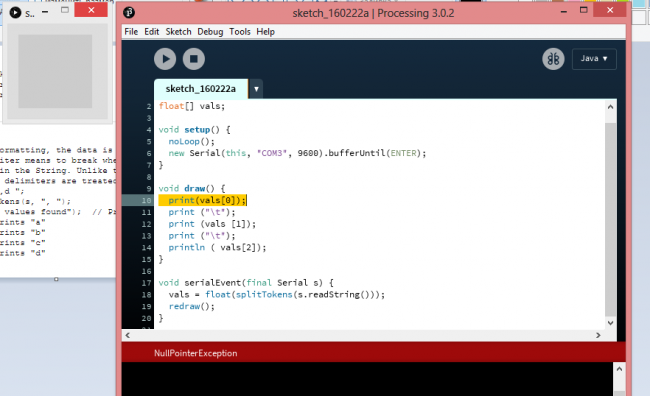#### Howdy, Stranger!

We are about to switch to a new forum software. Until then we have removed the registration on this forum.

# Drawing of graphs from I2C IMU

edited February 2016 in Arduino

Hello guys here is my code. I have tried to draw 3 graphs from arduino. I am reading angle by meaning inegration gyro angle , accelerometer angle and their complementary filter. But i get the message "Error, disabling serialEvent() for COM5 null"

``````import processing.serial.*;
Serial port;
float currentAngle ;
float pitchacc ;
float pitch ;
int gyro = 1;
int accel = 2;
int xn = 0;
float yn1 = 250;
float yn2 = 250;
float yn3 = 250;
int xk = 0;
void setup ()
{
size (500,500);
background (0);
port = new Serial ( this, "COM5", 9600);
port.bufferUntil ('\n');
}
void draw ()
{
fill(255);
stroke(255);
line (xn, yn1, xk, currentAngle);
line (xn, yn2, xk, pitchacc);
line (xn, yn3, xk, pitch);
if (xk > 500) {
background(0);
xk=0;
}
xn=xk;
yn1 = currentAngle;
yn2 = pitchacc;
yn3 = pitch;
xk++;
}

void serialEvent (Serial port)
{

}
``````
Tagged:

• ``````        currentAngle += gyroRate * (tt - t) / 1000.0;
Serial.print ( currentAngle);
Serial.print ("\t\t");
}
Serial.print (pitchacc);
Serial.print ("\t\t");
pitch = (pitchacc * 0.05 + 0.95 * currentAngle);
Serial.println (pitch);
``````

Here is my arduino code.

As I can guese I have something wrong with transforming data from string to float... in these 3 lines :

``````  currentAngle = float(port.readStringUntil(gyro));
``````
• Modify the Arduino's source to separate those sent values w/ `'\t'`:

``````// Forum.Processing.org/two/discussion/14988/drawing-of-graphs-from-i2c-imu#Item_3
// GoToLoop (2015-Feb-18)

import processing.serial.Serial;
float[] vals = {};

void setup() {
noLoop();
new Serial(this, "COM5", 9600).bufferUntil(ENTER);
}

void draw() {
println(vals);
}

void serialEvent(final Serial s) {
redraw();
}
``````
• If I understand correctly, I can print each value from String using " [ ] ", can't I? But when I try to execute " println (vals); " e.x., it says " NullPointerException'. For example if I need to build up 3 graphs I need to have 3 variables but I cannot use them separately.

• For my example to work, you need to modify how the data is sent from the device.
For example, if you send 3 `float` values: `println("10.5" + "\t" + "-2.3" + "\t" + "1.0");`

In Processing's end, it's expected: `vals = 10.5, vals = -2.3, vals = 1.0`

• I am just really confused. I understand how splitTokens operates and the rest. I can send to processing all data, and if I use your sketch it gives me such numbers : (see picture "example") But if I for example want to print only one of them ( e.x.  or ) it says me "NullPointerException"... May be I didn't understand correctly how to modify arduino's code may be something else... Here my adruino's code

``````#include <l3g4200d.h>
#include <lis331dlh.h>
#include <lis3mdl.h>
#include <LPS331.h>
#include <troyka-imu.h>
#include <Wire.h>
Gyroscope gyro;
Accelerometer accel;
float rotationThreshold = 1;   //Минимальная угловая скорость, которую можно не учитывать
float pitchacc;
float currentAngle;
float pitch;
boolean DR;
unsigned long t;
unsigned long tt;
void setup() {
Serial.begin (9600);
gyro.begin();
gyro.setRange(RANGE_500);
accel.begin();
DR = true;
pitch = 0;
}
void loop() {
// Тут только для первого лупа задаю начальное значие угла отклонения для гироскопа, который равен показанию акселерометра
if (DR = true)
{
t = micros();
DR = false;
}
tt = micros();
//Игнорировать показания гироскопа, если они меньше заданного лимита
if (gyroRate >= rotationThreshold || gyroRate <= -rotationThreshold) {
currentAngle += gyroRate * (tt - t) / 1000.0;
}
Serial.print(currentAngle);
Serial.print ("\t");
pitch = (pitchacc * 0.05 + 0.95 * currentAngle);
Serial.print (pitchacc);
Serial.print ("\t");
Serial.println ( pitch);
t = tt;
}
``````• Arduino code seems correct. Maybe show us how you're trying to get each individual value from vals[].

• Refering to splitToken reference explnation on Processing website, I want to get all the value independently and use them as y - coordinate for graphs building.• edited February 2016 Answer ✓

Until serialEvent() is finally called back for the 1st time, vals is still `null`!
An easy fix is simply instantiating some `float[]` array at the same time vals is declared:
Change: `float[] vals;` to `float[] vals = new float;`

• Perfect! Now it is what I want! Appreciate it! One moment, could you please describe a bit how `float [] vals = new float;` works? I have read on website, but still it is not absoulutelly clear for me. Thanks!

• Processing always runs draw() at least once, even w/ noLoop() active.
• If we only declared variable vals, it's still `null` when it reaches `println(vals);`.
• Logically `vals` crashes b/c we can't do anything w/ `null` but print it raw: `println(vals);`
• However, by initializing vals w/ an arbitrary `new float`, it's not `null` anymore.
• And it's now safe for `println(vals);`. Although it's just gonna display `0.0` at that 1st draw().
• But once serialEvent() gets triggered, vals is re-assigned w/ the correct `float[]` array generated by `float(splitTokens(s.readString()));` expression. :-bd
• And then redraw() requests draw() to run once, displaying the correct received data from now on.
• ``````void draw() {
println(vals, TAB, vals, TAB, vals);
}
``````